# Introduction to RxODE

## RxODE: A tool for performing simulations from Ordinary Differential Equation (ODE) models, with applications for pharmacometrics

#### Introduction

RxODE is an R package that facilitates simulation with ODE models in R. It is designed with pharmacometrics models in mind, but can be applied more generally to any ODE model.

#### Description of RxODE illustrated through an example

The model equations are specified through a text string in R. Both differential and algebraic equations are permitted. Differential equations are specified by d/dt(var_name) =. Each equation can be separated by a semicolon.

ode <- "
C2 = centr/V2;
C3 = peri/V3;
d/dt(depot) =-KA*depot;
d/dt(centr) = KA*depot - CL*C2 - Q*C2 + Q*C3;
d/dt(peri)  =                    Q*C2 - Q*C3;
d/dt(eff)  = Kin - Kout*(1-C2/(EC50+C2))*eff;
"

To load RxODE package and compile the model:

library(RxODE)
work <- tempfile("Rx_intro-")
mod1 <- RxODE(model = ode, modName = "mod1", wd = work)
##### Specify ODE parameters and initial conditions

Model parameters can be defined as named vectors. Names of parameters in the vector must be a superset of parameters in the ODE model, and the order of parameters within the vector is not important.

theta <-
c(KA=2.94E-01, CL=1.86E+01, V2=4.02E+01, # central
Q=1.05E+01,  V3=2.97E+02,              # peripheral
Kin=1, Kout=1, EC50=200)               # effects

Initial conditions (ICs) are defined through a vector as well. If the vector is not named, the number of ICs must equal exactly the number of ODEs in the model, and the order must be the same as the order in which the ODEs are listed in the model.

inits <- c(0, 0, 0, 1)

When elements are named, missing elements are added and set to zero. Also when named, the order of initilizations does not matter. Therefore the following code is an equvalent initialization as the code above.

inits <- c(eff=1);
##### Specify Dosing and sampling in RxODE

RxODE provides a simple and very flexible way to specify dosing and sampling through functions that generate an event table. First, an empty event table is generated through the “eventTable()” function:

ev <- eventTable(amount.units='mg', time.units='hours')

Next, use the add.dosing() and add.sampling() functions of the EventTable object to specify the dosing (amounts, frequency and/or times, etc.) and observation times at which to sample the state of the system. These functions can be called multiple times to specify more complex dosing or sampling regiments. Here, these functions are used to specify 10mg BID dosing for 5 days, followed by 20mg QD dosing for 5 days:

ev$add.dosing(dose=10000, nbr.doses=10, dosing.interval=12) ev$add.dosing(dose=20000, nbr.doses=5, start.time=120, dosing.interval=24)
ev$add.sampling(0:240) If you wish you can also do this with the mattigr pipe operator %>% ev <- eventTable(amount.units="mg", time.units="hours") %>% add.dosing(dose=10000, nbr.doses=10, dosing.interval=12) %>% add.dosing(dose=20000, nbr.doses=5, start.time=120,dosing.interval=24) %>% add.sampling(0:240); The functions get.dosing() and get.sampling() can be used to retrieve information from the event table. head(ev$get.dosing())
##   time evid   amt
## 1    0  101 10000
## 2   12  101 10000
## 3   24  101 10000
## 4   36  101 10000
## 5   48  101 10000
## 6   60  101 10000
head(ev$get.sampling()) ## time evid amt ## 16 0 0 NA ## 17 1 0 NA ## 18 2 0 NA ## 19 3 0 NA ## 20 4 0 NA ## 21 5 0 NA ##### Solving ODEs The ODE can now be solved by calling the model object’s run or solve function. Simulation results for all variables in the model are stored in the output matrix x. x <- mod1$solve(theta, ev, inits)
head(x)
##      time     depot    centr      peri      eff       C2        C3
## [1,]    0 10000.000    0.000    0.0000 1.000000  0.00000 0.0000000
## [2,]    1  7452.765 1783.897  273.1895 1.084664 44.37555 0.9198298
## [3,]    2  5554.370 2206.295  793.8758 1.180825 54.88296 2.6729825
## [4,]    3  4139.542 2086.518 1323.5783 1.228914 51.90343 4.4564927
## [5,]    4  3085.103 1788.795 1776.2702 1.234610 44.49738 5.9807076
## [6,]    5  2299.255 1466.670 2131.7169 1.214742 36.48434 7.1774981

This returns a matrix. You can see the compartment values in the plot below:

par(mfrow=c(1,2))
matplot(x[,"C2"], type="l", ylab="Central Concentration")
matplot(x[,"eff"], type="l", ylab = "Effect")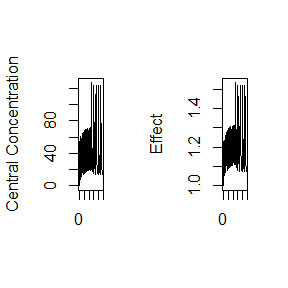##### Using RxODE data frames

You can also return a solved object that is a modified data-frame. This is done by the predict() or solve() methods:

x <- predict(mod1,theta, ev, inits)
print(x)
## Solved RxODE object
## Dll: C:\Users\fidlema3\AppData\Local\Temp\ep\RtmpgHLtwg\Rx_intro-37a078a95b4a/mod1.d/mod1_x64.dll
##
## Parameters:
##      V2      V3      KA      CL       Q     Kin    Kout    EC50
##  40.200 297.000   0.294  18.600  10.500   1.000   1.000 200.000
##
##
## Initial Conditions:
## depot centr  peri   eff
##     0     0     0     1
##
##
## First part of data:
## # A tibble: 241 x 7
##    time     depot    centr      peri      eff       C2        C3
##   <dbl>     <dbl>    <dbl>     <dbl>    <dbl>    <dbl>     <dbl>
## 1     0 10000.000    0.000    0.0000 1.000000  0.00000 0.0000000
## 2     1  7452.765 1783.897  273.1895 1.084664 44.37555 0.9198298
## 3     2  5554.370 2206.295  793.8758 1.180825 54.88296 2.6729825
## 4     3  4139.542 2086.518 1323.5783 1.228914 51.90343 4.4564927
## 5     4  3085.103 1788.795 1776.2702 1.234610 44.49738 5.9807076
## 6     5  2299.255 1466.670 2131.7169 1.214742 36.48434 7.1774981
## # ... with 235 more rows

or

x <- solve(mod1,theta, ev, inits)
print(x)
## Solved RxODE object
## Dll: C:\Users\fidlema3\AppData\Local\Temp\ep\RtmpgHLtwg\Rx_intro-37a078a95b4a/mod1.d/mod1_x64.dll
##
## Parameters:
##      V2      V3      KA      CL       Q     Kin    Kout    EC50
##  40.200 297.000   0.294  18.600  10.500   1.000   1.000 200.000
##
##
## Initial Conditions:
## depot centr  peri   eff
##     0     0     0     1
##
##
## First part of data:
## # A tibble: 241 x 7
##    time     depot    centr      peri      eff       C2        C3
##   <dbl>     <dbl>    <dbl>     <dbl>    <dbl>    <dbl>     <dbl>
## 1     0 10000.000    0.000    0.0000 1.000000  0.00000 0.0000000
## 2     1  7452.765 1783.897  273.1895 1.084664 44.37555 0.9198298
## 3     2  5554.370 2206.295  793.8758 1.180825 54.88296 2.6729825
## 4     3  4139.542 2086.518 1323.5783 1.228914 51.90343 4.4564927
## 5     4  3085.103 1788.795 1776.2702 1.234610 44.49738 5.9807076
## 6     5  2299.255 1466.670 2131.7169 1.214742 36.48434 7.1774981
## # ... with 235 more rows

Or with mattigr

x <- mod1 %>% solve(theta, ev, inits)
print(x)
## Solved RxODE object
## Dll: C:\Users\fidlema3\AppData\Local\Temp\ep\RtmpgHLtwg\Rx_intro-37a078a95b4a/mod1.d/mod1_x64.dll
##
## Parameters:
##      V2      V3      KA      CL       Q     Kin    Kout    EC50
##  40.200 297.000   0.294  18.600  10.500   1.000   1.000 200.000
##
##
## Initial Conditions:
## depot centr  peri   eff
##     0     0     0     1
##
##
## First part of data:
## # A tibble: 241 x 7
##    time     depot    centr      peri      eff       C2        C3
##   <dbl>     <dbl>    <dbl>     <dbl>    <dbl>    <dbl>     <dbl>
## 1     0 10000.000    0.000    0.0000 1.000000  0.00000 0.0000000
## 2     1  7452.765 1783.897  273.1895 1.084664 44.37555 0.9198298
## 3     2  5554.370 2206.295  793.8758 1.180825 54.88296 2.6729825
## 4     3  4139.542 2086.518 1323.5783 1.228914 51.90343 4.4564927
## 5     4  3085.103 1788.795 1776.2702 1.234610 44.49738 5.9807076
## 6     5  2299.255 1466.670 2131.7169 1.214742 36.48434 7.1774981
## # ... with 235 more rows

The solved object acts as a data.frame or tbl that can be filtered by dpylr. For example you could filter it easily.

library(dplyr)
##
## Attaching package: 'dplyr'
## The following objects are masked from 'package:stats':
##
##     filter, lag
## The following objects are masked from 'package:base':
##
##     intersect, setdiff, setequal, union
x <- mod1 %>% solve(theta,ev,inits) %>%  filter(time <=3)
x
##   time     depot    centr      peri      eff       C2        C3
## 1    0 10000.000    0.000    0.0000 1.000000  0.00000 0.0000000
## 2    1  7452.765 1783.897  273.1895 1.084664 44.37555 0.9198298
## 3    2  5554.370 2206.295  793.8758 1.180825 54.88296 2.6729825
## 4    3  4139.542 2086.518 1323.5783 1.228914 51.90343 4.4564927

However it isn’t just a simple data object. You can use the solved object to update paramters on the fly, or even change the sampling time.

First we need to recreate the original solved system:

x <- mod1 %>% solve(theta,ev,inits);

To examine or change initial conditions, you can use the syntax cmt.0, cmt0, or cmt_0. In the case of the eff compartment defined by the model, this is:

x$eff0 ## eff ## 1 which shows the initial condition of the effect compartment. If you wished to change this initial condition to 2, this can be done easily by: x$eff0 <- 2
## Updating object with new initial conditions.
x
## Solved RxODE object
## Dll: C:\Users\fidlema3\AppData\Local\Temp\ep\RtmpgHLtwg\Rx_intro-37a078a95b4a/mod1.d/mod1_x64.dll
##
## Parameters:
##      V2      V3      KA      CL       Q     Kin    Kout    EC50
##  40.200 297.000   0.294  18.600  10.500   1.000   1.000 200.000
##
##
## Initial Conditions:
## depot centr  peri   eff
##     0     0     0     2
##
##
## First part of data:
## # A tibble: 241 x 7
##    time     depot    centr      peri      eff       C2        C3
##   <dbl>     <dbl>    <dbl>     <dbl>    <dbl>    <dbl>     <dbl>
## 1     0 10000.000    0.000    0.0000 2.000000  0.00000 0.0000000
## 2     1  7452.765 1783.897  273.1895 1.496778 44.37555 0.9198299
## 3     2  5554.370 2206.295  793.8759 1.366782 54.88295 2.6729829
## 4     3  4139.542 2086.517 1323.5786 1.313536 51.90341 4.4564937
## 5     4  3085.103 1788.793 1776.2706 1.272430 44.49735 5.9807092
## 6     5  2299.255 1466.669 2131.7173 1.231204 36.48431 7.1774995
## # ... with 235 more rows

Notice that the inital effect is now 2.

You can also change the sampling times easily by this method by changing t or time. For example:

x$t <- seq(0,5,length.out=20) ## Updating sampling times in the event table updating object. x ## Solved RxODE object ## Dll: C:\Users\fidlema3\AppData\Local\Temp\ep\RtmpgHLtwg\Rx_intro-37a078a95b4a/mod1.d/mod1_x64.dll ## ## Parameters: ## V2 V3 KA CL Q Kin Kout EC50 ## 40.200 297.000 0.294 18.600 10.500 1.000 1.000 200.000 ## ## ## Initial Conditions: ## depot centr peri eff ## 0 0 0 2 ## ## ## First part of data: ## # A tibble: 20 x 7 ## time depot centr peri eff C2 C3 ## <dbl> <dbl> <dbl> <dbl> <dbl> <dbl> <dbl> ## 1 0.0000000 10000.000 0.0000 0.00000 2.000000 0.00000 0.0000000 ## 2 0.2631579 9255.488 677.1371 24.26134 1.787179 16.84421 0.0816880 ## 3 0.5263158 8566.406 1186.8059 88.67937 1.646825 29.52253 0.2985837 ## 4 0.7894737 7928.627 1562.0986 182.59671 1.551517 38.85817 0.6148038 ## 5 1.0526316 7338.331 1830.0078 297.50288 1.485338 45.52258 1.0016932 ## 6 1.3157895 6791.983 2012.5211 426.63581 1.438438 50.06271 1.4364842 ## # ... with 14 more rows You can also access or change parameters by the $ operator. For example, accessing KA can be done by:

x$KA ## KA ## 0.294 And you may change it by assigning it to a new value. x$KA <- 1;
## Updating object with new parameter values.
x
## Solved RxODE object
## Dll: C:\Users\fidlema3\AppData\Local\Temp\ep\RtmpgHLtwg\Rx_intro-37a078a95b4a/mod1.d/mod1_x64.dll
##
## Parameters:
##    V2    V3    KA    CL     Q   Kin  Kout  EC50
##  40.2 297.0   1.0  18.6  10.5   1.0   1.0 200.0
##
##
## Initial Conditions:
## depot centr  peri   eff
##     0     0     0     2
##
##
## First part of data:
## # A tibble: 20 x 7
##        time     depot    centr       peri      eff        C2        C3
##       <dbl>     <dbl>    <dbl>      <dbl>    <dbl>     <dbl>     <dbl>
## 1 0.0000000 10000.000    0.000    0.00000 2.000000   0.00000 0.0000000
## 2 0.2631579  7686.205 2098.224   77.62338 1.822345  52.19463 0.2613582
## 3 0.5263158  5907.775 3348.269  267.29379 1.737850  83.29027 0.8999791
## 4 0.7894737  4540.837 4010.290  519.32777 1.692658  99.75845 1.7485784
## 5 1.0526316  3490.181 4272.980  799.73120 1.665007 106.29303 2.6926976
## 6 1.3157895  2682.625 4272.092 1085.82977 1.644283 106.27096 3.6559925
## # ... with 14 more rows

You can access/change all the parametrs, initilizations or events with the $params, $inits, $events accessor syntax, similar to what is used above. This syntax makes it easy to update and explore the effect of various parameters on the solved object. #### Mixing Solved Systems and ODEs. In addition to pure ODEs, you may mix solved systems and ODEs. The prior 2-compartment indirect response model can be simplified with a linCmt() function: mod2 <- RxODE({ ## the order of variables do not matter, the type of compartmental ## model is determined by the parameters specified. C2 = linCmt(KA, CL, V2, Q, V3); eff(0) = 1 ## This specifies that the effect compartment starts at 1. d/dt(eff) = Kin - Kout*(1-C2/(EC50+C2))*eff; }) Like a Sherlock Holmes on the case of a mystery, the linCmt() function figures out the type of model to use based on the parameter names specified. Most often, pharmacometric models are parameterized in terms of volume and clearances. Clearances are specified by NONMEM-style names of CL, Q, Q1, Q2, etc. or distributional clearances CLD, CLD2. Volumes are specified by Central (VC or V), Peripheral/Tissue (VP, VT). Another popular parameterization is in terms of micro-constants. RxODE assumes compartment 1 is the central compartment. The elimination constant would be specified by K, Ke or Kel. Once the linCmt() sleuthing is complete, the 1, 2 or 3 compartment model solution is used as the value of linCmt(). This allows the indirect response model above to assign the 2-compartment model to the C2 variable and the used in the indirect response model. When mixing the solved systems and the ODEs, the solved system’s compartment is always the last compartment. This is because the solved system technically isn’t a compartment to be solved. Adding the dosing compartment to the end will not interfere with the actual ODE to be solved. Therefore,in the two-compartment indirect response model, the effect compartment is compartment #1 while the PK dosing compartment for the depot is compartment #2. This compartment model requires a new event table since the compartment number changed: ev <- eventTable(amount.units='mg', time.units='hours') %>% add.dosing(dose=10000, nbr.doses=10, dosing.interval=12,dosing.to=2) %>% add.dosing(dose=20000, nbr.doses=5, start.time=120,dosing.interval=24,dosing.to=2) %>% add.sampling(0:240); This can be solved with the following command: (x <- mod2 %>% solve(theta, ev)) ## Solved RxODE object ## Dll: C:/Users/fidlema3/AppData/Local/Temp/ep/Rtmp2pDK5l/Rbuild311c418e6c4d/RxODE/vignettes/rx_cc5428ef72de11dcf799b881dee6547a_x64.dll ## ## Parameters: ## KA V2 CL Q V3 Kin Kout EC50 ## 0.294 40.200 18.600 10.500 297.000 1.000 1.000 200.000 ## ## ## Initial Conditions: ## eff ## 1 ## ## ## First part of data: ## # A tibble: 241 x 3 ## time eff C2 ## <dbl> <dbl> <dbl> ## 1 0 1.000000 0.00000 ## 2 1 1.084665 44.37555 ## 3 2 1.180826 54.88295 ## 4 3 1.228914 51.90342 ## 5 4 1.234610 44.49737 ## 6 5 1.214743 36.48434 ## # ... with 235 more rows Note this solving did not require specifying the effect compartment initial condition to be 1. Rather, this is already pre-specified by eff(0)=1. This can be solved for different initial conditions easily: (x <- mod2 %>% solve(theta, ev,c(eff=2))) ## Solved RxODE object ## Dll: C:/Users/fidlema3/AppData/Local/Temp/ep/Rtmp2pDK5l/Rbuild311c418e6c4d/RxODE/vignettes/rx_cc5428ef72de11dcf799b881dee6547a_x64.dll ## ## Parameters: ## KA V2 CL Q V3 Kin Kout EC50 ## 0.294 40.200 18.600 10.500 297.000 1.000 1.000 200.000 ## ## ## Initial Conditions: ## eff ## 2 ## ## ## First part of data: ## # A tibble: 241 x 3 ## time eff C2 ## <dbl> <dbl> <dbl> ## 1 0 2.000000 0.00000 ## 2 1 1.496778 44.37555 ## 3 2 1.366782 54.88295 ## 4 3 1.313536 51.90342 ## 5 4 1.272430 44.49737 ## 6 5 1.231204 36.48434 ## # ... with 235 more rows The RxODE detective also does not require you to specify the variables in the linCmt() function if they are already defined in the block. Therefore, the following function will also work to solve the same system. mod3 <- RxODE({ KA=2.94E-01; CL=1.86E+01; V2=4.02E+01; Q=1.05E+01; V3=2.97E+02; Kin=1; Kout=1; EC50=200; ## The linCmt() picks up the variables from above C2 = linCmt(); eff(0) = 1 ## This specifies that the effect compartment starts at 1. d/dt(eff) = Kin - Kout*(1-C2/(EC50+C2))*eff; }) (x <- mod3 %>% solve(ev)) ## Solved RxODE object ## Dll: C:/Users/fidlema3/AppData/Local/Temp/ep/Rtmp2pDK5l/Rbuild311c418e6c4d/RxODE/vignettes/rx_a96ab6c393837232ef0776245b3c42a0_x64.dll ## ## Parameters: ## KA CL V2 Q V3 Kin Kout EC50 ## 0.294 18.600 40.200 10.500 297.000 1.000 1.000 200.000 ## ## ## Initial Conditions: ## eff ## 1 ## ## ## First part of data: ## # A tibble: 241 x 3 ## time eff C2 ## <dbl> <dbl> <dbl> ## 1 0 1.000000 0.00000 ## 2 1 1.084665 44.37555 ## 3 2 1.180826 54.88295 ## 4 3 1.228914 51.90342 ## 5 4 1.234610 44.49737 ## 6 5 1.214743 36.48434 ## # ... with 235 more rows Note that you do not specify the parameters when solving the system since they are built into the model, but you can override the parameters: (x <- mod3 %>% solve(c(KA=10),ev)) ## Solved RxODE object ## Dll: C:/Users/fidlema3/AppData/Local/Temp/ep/Rtmp2pDK5l/Rbuild311c418e6c4d/RxODE/vignettes/rx_a96ab6c393837232ef0776245b3c42a0_x64.dll ## ## Parameters: ## KA CL V2 Q V3 Kin Kout EC50 ## 10.0 18.6 40.2 10.5 297.0 1.0 1.0 200.0 ## ## ## Initial Conditions: ## eff ## 1 ## ## ## First part of data: ## # A tibble: 241 x 3 ## time eff C2 ## <dbl> <dbl> <dbl> ## 1 0 1.000000 0.00000 ## 2 1 1.340982 130.61937 ## 3 2 1.392185 64.75317 ## 4 3 1.298397 33.17930 ## 5 4 1.191799 18.02218 ## 6 5 1.115887 10.72199 ## # ... with 235 more rows #### ODEs and covariates Covariates are easy to specify in RxODE, you can specify them as a variable. Time-varying covariates, like clock time in a circadian rhythm model, can also be used. Extending the indirect response model already discussed, we have: mod3 <- RxODE({ KA=2.94E-01; CL=1.86E+01; V2=4.02E+01; Q=1.05E+01; V3=2.97E+02; Kin0=1; Kout=1; EC50=200; ## The linCmt() picks up the variables from above C2 = linCmt(); Tz= 8 amp=0.1 eff(0) = 1 ## This specifies that the effect compartment starts at 1. ## Kin changes based on time of day (like cortosol) Kin = Kin0 +amp *cos(2*pi*(ctime-Tz)/24) d/dt(eff) = Kin - Kout*(1-C2/(EC50+C2))*eff; }) ev <- eventTable(amount.units="mg", time.units="hours") %>% add.dosing(dose=10000, nbr.doses=1, dosing.to=2) %>% add.sampling(seq(0,48,length.out=100)); ## Create data frame of 8 am dosing for the first dose cov.df <- data.frame(ctime =(seq(0,48,length.out=100)+8) %% 24); Now there is a covariate present, the system can be solved using the cov option (r1 <- solve(mod3, ev, covs=cov.df,covs_interpolation="linear")) ## Solved RxODE object ## Dll: C:/Users/fidlema3/AppData/Local/Temp/ep/Rtmp2pDK5l/Rbuild311c418e6c4d/RxODE/vignettes/rx_4780fbffa6b2d111771a4be0ab39df5f_x64.dll ## ## Parameters: ## KA CL V2 Q V3 Kin0 ## 0.294000 18.600000 40.200000 10.500000 297.000000 1.000000 ## Kout EC50 Tz amp pi ## 1.000000 200.000000 8.000000 0.100000 3.141593 ## ## Time Varying Covariates ## ctime ## ## ## Initial Conditions: ## eff ## 1 ## ## ## First part of data: ## # A tibble: 100 x 4 ## time eff C2 Kin ## <dbl> <dbl> <dbl> <dbl> ## 1 0.0000000 1.000000 0.00000 1.099195 ## 2 0.4848485 1.067175 27.76574 1.096795 ## 3 0.9696970 1.144556 43.67518 1.092837 ## 4 1.4545455 1.212373 51.75572 1.087385 ## 5 1.9393939 1.263980 54.76289 1.080527 ## 6 2.4242424 1.298023 54.57072 1.072373 ## # ... with 94 more rows When solving ODE equations, the solver may sample times outside of the data. When this happens, this ODE solver uses linear interpolation between the covariate values. This is the default value. It is equivalent to R’s approxfun with method="linear", which is the default approxfun. par(mfrow=c(1,2)) matplot(r1[,"C2"], type="l", ylab="Central Concentration") matplot(r1[,"eff"], type="l", ylab = "Effect")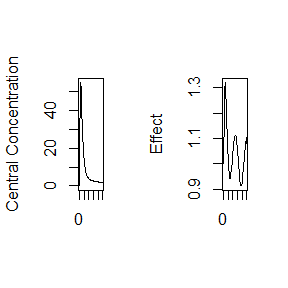Note that the linear approximation in this case leads to some kinks in the solved system at 24-hours where the covariate has a linear interpolation between near 24 and near 0. In RxODE, covariate interpolation can also be the last observation carried forward, or constant approximation. This is equivalent to R’s approxfun with method="constant". (r2 <- solve(mod3, ev, covs=cov.df,covs_interpolation="constant")) ## Solved RxODE object ## Dll: C:/Users/fidlema3/AppData/Local/Temp/ep/Rtmp2pDK5l/Rbuild311c418e6c4d/RxODE/vignettes/rx_4780fbffa6b2d111771a4be0ab39df5f_x64.dll ## ## Parameters: ## KA CL V2 Q V3 Kin0 ## 0.294000 18.600000 40.200000 10.500000 297.000000 1.000000 ## Kout EC50 Tz amp pi ## 1.000000 200.000000 8.000000 0.100000 3.141593 ## ## Time Varying Covariates ## ctime ## ## ## Initial Conditions: ## eff ## 1 ## ## ## First part of data: ## # A tibble: 100 x 4 ## time eff C2 Kin ## <dbl> <dbl> <dbl> <dbl> ## 1 0.0000000 1.000000 0.00000 1.099195 ## 2 0.4848485 1.067628 27.76574 1.096795 ## 3 0.9696970 1.145644 43.67518 1.092837 ## 4 1.4545455 1.214226 51.75572 1.087385 ## 5 1.9393939 1.266668 54.76289 1.080527 ## 6 2.4242424 1.301568 54.57072 1.072373 ## # ... with 94 more rows which dives the following plots: par(mfrow=c(1,2)) matplot(r2[,"C2"], type="l", ylab="Central Concentration") matplot(r2[,"eff"], type="l", ylab = "Effect")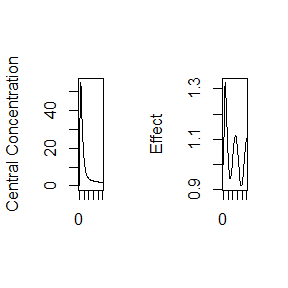In this case, the plots seem to be smoother. #### RxODE and transit compartment models Savic 2008 first introduced the idea of transit compartments being a mechanistic explanation of a a lag-time type phenomena. RxODE has special handling of these models: You can specify this in a similar manner as the original paper: mod <- RxODE({ ## Table 3 from Savic 2007 cl = 17.2 # (L/hr) vc = 45.1 # L ka = 0.38 # 1/hr mtt = 0.37 # hr bio=1 n = 20.1 k = cl/vc ktr = (n+1)/mtt ## note that lgammafn is the same as lgamma in R. d/dt(depot) = exp(log(bio*podo)+log(ktr)+n*log(ktr*t)-ktr*t-lgammafn(n+1))-ka*depot d/dt(cen) = ka*depot-k*cen }) et <- eventTable(); et$add.sampling(seq(0, 7, length.out=200));
et$add.dosing(20, start.time=0); transit <- rxSolve(mod, et, transit_abs=TRUE) par(mfrow=c(1,1)) with(transit,matplot(time,cen, type="l", ylab="Central Concentration", xlab=""))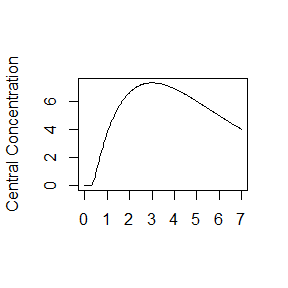Another option is to specify the transit compartment function transit syntax. This specifies the parameters transit(number of transit compartments, mean transit time, bioavailibility). The bioavailibity term is optional. Using the transit code also automatically turns on the transit_abs option. Therefore, the same model can be specified by: mod <- RxODE({ ## Table 3 from Savic 2007 cl = 17.2 # (L/hr) vc = 45.1 # L ka = 0.38 # 1/hr mtt = 0.37 # hr bio=1 n = 20.1 k = cl/vc ktr = (n+1)/mtt d/dt(depot) = transit(n,mtt,bio)-ka*depot d/dt(cen) = ka*depot-k*cen }) et <- eventTable(); et$add.sampling(seq(0, 7, length.out=200));
et$add.dosing(20, start.time=0); transit <- rxSolve(mod, et) ## Warning in object$solve(params, events, inits, scale, covs, stiff,
## transit_abs, : Assumed transit compartment model since 'podo' is in the
## model.
par(mfrow=c(1,1))
with(transit,matplot(time,cen, type="l", ylab="Central Concentration", xlab=""))#### Stiff ODEs with Jacobian Specification

Occasionally, you may come across a stiff differential equation, that is a differential equation that is numerically unstable and small variations in parameters cause different solutions to the ODEs. One way to tackle this is to choose a stiff-solver, or hybrid stiff solver (like the default LSODA). Typically this is enough. However exact Jacobian solutions may increase the stability of the ODE. (Note the Jacobian is the derivative of the ODE specification with respect to each variable). In RxODE you can specify the Jacobian with the df(state)/dy(variable)= statement. A classic ODE that has stiff properties under various conditions is the van dar Pol differential equations.

In RxODE these can be specified by the following:

Vtpol2 <- RxODE({
d/dt(y)  = dy
d/dt(dy) = mu*(1-y^2)*dy - y
## Jacobian
df(y)/dy(dy)  = 1
df(dy)/dy(y)  = -2*dy*mu*y - 1
df(dy)/dy(dy) = mu*(1-y^2)
## Initial conditions
y(0) = 2
dy(0) = 0
## mu
mu = 1 ## nonstiff; 10 moderately stiff; 1000 stiff
})

et <- eventTable();
et$add.sampling(seq(0, 10, length.out=200)); et$add.dosing(20, start.time=0);

(s1 <- Vtpol2 %>%  solve(et))
## Solved RxODE object
## Dll: C:/Users/fidlema3/AppData/Local/Temp/ep/Rtmp2pDK5l/Rbuild311c418e6c4d/RxODE/vignettes/rx_2257a2a61f22998cf71203eb769025e5_x64.dll
##
## Parameters:
## mu
##  1
##
##
## Initial Conditions:
##  y dy
##  2  0
##
##
## First part of data:
## # A tibble: 200 x 3
##         time        y          dy
##        <dbl>    <dbl>       <dbl>
## 1 0.00000000 22.00000  0.00000000
## 2 0.05025126 21.99781 -0.04555302
## 3 0.10050251 21.99552 -0.04555778
## 4 0.15075377 21.99323 -0.04556254
## 5 0.20100503 21.99094 -0.04556731
## 6 0.25125628 21.98865 -0.04557207
## # ... with 194 more rows

While this is not stiff at mu=1, mu=1000 is a stiff system

(s2 <- Vtpol2 %>%  solve(c(mu=1000), et))
## Solved RxODE object
## Dll: C:/Users/fidlema3/AppData/Local/Temp/ep/Rtmp2pDK5l/Rbuild311c418e6c4d/RxODE/vignettes/rx_2257a2a61f22998cf71203eb769025e5_x64.dll
##
## Parameters:
##   mu
## 1000
##
##
## Initial Conditions:
##  y dy
##  2  0
##
##
## First part of data:
## # A tibble: 200 x 3
##         time        y            dy
##        <dbl>    <dbl>         <dbl>
## 1 0.00000000 22.00000  0.000000e+00
## 2 0.05025126 22.00000 -4.554866e-05
## 3 0.10050251 22.00000 -4.554866e-05
## 4 0.15075377 21.99999 -4.554867e-05
## 5 0.20100503 21.99999 -4.554867e-05
## 6 0.25125628 21.99999 -4.554868e-05
## # ... with 194 more rows

While this is easy enough to do, it is a bit tedious. If you have RxODE setup appropriately, that is you have:

• Python installed in your system
• sympy installed in your system
• SnakeCharmR installed in R

You can use the computer algebra system sympy to calculate the Jacobian automatically.

This is done by the RxODE option calcJac option:

Vtpol <- RxODE({
d/dt(y)  = dy
d/dt(dy) = mu*(1-y^2)*dy - y
## Initial conditions
y(0) = 2
dy(0) = 0
## mu
mu = 1 ## nonstiff; 10 moderately stiff; 1000 stiff
}, calcJac=TRUE)


To see the generated model, you can use rxCat:

> rxCat(Vtpol)
d/dt(y)=dy;
d/dt(dy)=mu*(1-y^2)*dy-y;
y(0)=2;
dy(0)=0;
mu=1;
df(y)/dy(y)=0;
df(dy)/dy(y)=-2*dy*mu*y-1;
df(y)/dy(dy)=1;
df(dy)/dy(dy)=mu*(-Rx_pow_di(y,2)+1);


#### Simulation of Variability with RxODE

Variability in model parameters can be simulated by creating a matrix of parameter values for use in the simulation. In the example below, 40% variability in clearance is simulated.

nsub <- 100                       #number of subproblems
CL <- 1.86E+01*exp(rnorm(nsub,0,.4^2))
theta.all <-
cbind(KA=2.94E-01, CL=CL, V2=4.02E+01,  # central
Q=1.05E+01, V3=2.97E+02,                # peripheral
Kin=1, Kout=1, EC50=200)                # effects
head(theta.all)
##         KA       CL   V2    Q  V3 Kin Kout EC50
## [1,] 0.294 16.69295 40.2 10.5 297   1    1  200
## [2,] 0.294 20.04386 40.2 10.5 297   1    1  200
## [3,] 0.294 19.21716 40.2 10.5 297   1    1  200
## [4,] 0.294 20.87767 40.2 10.5 297   1    1  200
## [5,] 0.294 25.19626 40.2 10.5 297   1    1  200
## [6,] 0.294 15.96079 40.2 10.5 297   1    1  200

Each subproblem can be simulated by using an explicit loop (or the apply() function) to run the simulation for each set of parameters of in the parameter matrix.

nobs <- ev$get.nobs() set.seed(1) cp.all <- matrix(NA, nobs, nsub) for (i in 1:nsub) { theta <- theta.all[i,] x <- mod1$solve(theta, ev, inits=inits)
cp.all[, i] <- x[, "C2"]
}

matplot(cp.all, type="l", ylab="Central Concentration")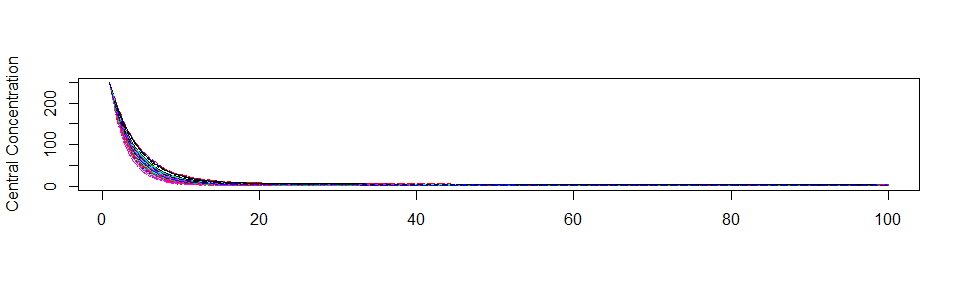It is now straightforward to perform calculations and generate plots with the simulated data. Below, the 5th, 50th, and 95th percentiles of the simulated data are plotted.

cp.q <- apply(cp.all, 1, quantile, prob = c(0.05, 0.50, 0.95))
matplot(t(cp.q), type="l", lty=c(2,1,2), col=c(2,1,2), ylab="Central Concentration")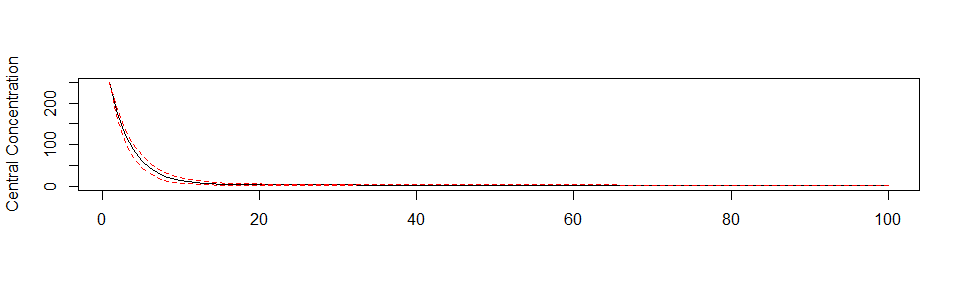#### Facilities for generating R shiny applications

An example of creating an R shiny application to interactively explore responses of various complex dosing regimens is available at http://qsp.engr.uga.edu:3838/RxODE/RegimenSimulator. Shiny applications like this one may be programmatically created with the experimental function genShinyApp.template().

The above application includes widgets for varying the dose, dosing regimen, dose cycle, and number of cycles.


genShinyApp.template(appDir = "shinyExample", verbose=TRUE)

library(shiny)
runApp("shinyExample")